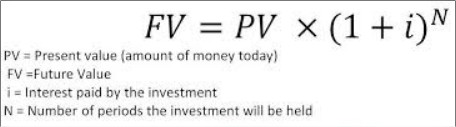# Java Program To Calculate Future Investment Value

Java programs to calculate the future investment value – In this particular article, we will brief in on the various ways to calculate the future investment value in Java Programming.

Suitable examples and sample programs are added for the better understanding of the people who are interested in this specific article. The compiler has also been added where you can execute the programs by yourself.

The methods used in this particular piece are as follows:

• Taking Input From Scanner Class
• Taking Input From Command-line Arguments
• Using Separate “fiv” Calculation class
• Using Static Function

Future Investment Value or simply, Future Value is the worth of an asset at a given point in time. The value that determines the value at that particular time period are:

• Interest Rate or
• Rate of ReturnAs you can see, this is the formula for calculating the Future Investment Value. PV is known as the Present Value or simply the Principal.

The (1 + i)^n is regarded as the Accumulation function which determines the appreciation or depreciation of the said asset.

“i” = The interest paid by the investment.

“n” or “y” = the time in years the investment is held.

Thus, the methods used to calculate the Future Investment Value in Java Programming are as follows:

Output:

Output:

Output:
Output:
x

## Plus Star Pattern Java Program | Patterns

Java program to print plus star pattern program – We have written the below print/draw ...